Bonky Zhu
If someone is able to show me that what I think or do is not right, I will happily change, for I seek the truth, by which no one was ever truly harmed. It is the person who continues in his self-deception and ignorance who is harmed.

Pytorch 的自动求导机制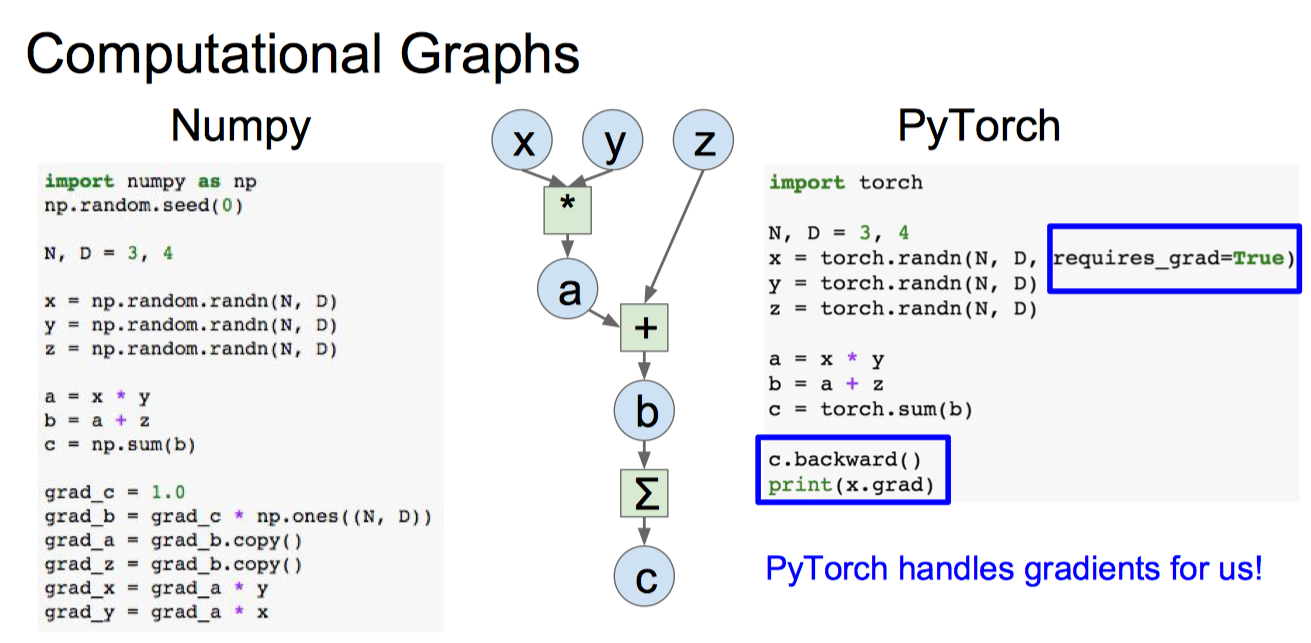简介

>>> x = torch.ones(2,4,requires_grad=True) >>> y = x * 5 >>> y tensor([[5., 5., 5., 5.],         [5., 5., 5., 5.]], grad_fn=)
True

>>> y.requires_grad_(False) Traceback (most recent call last):   File "", line 1, in
RuntimeError: you can only change requires_grad flags of leaf variables. If you want to use a computed variable in a subgraph that doesnt require differentiation use var_no_grad = var.detach().

Pytorch的计算图

构建一个计算图

pytorch是动态图机制，所以在训练模型时候，每迭代一次都会构建一个新的计算图。而计算图其实就是代表程序中变量之间的关系。举个列子：(b%2Bc)) 在这个运算过程就会建立一个如下的计算图：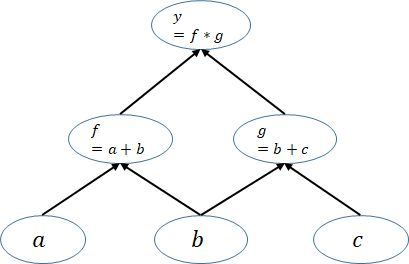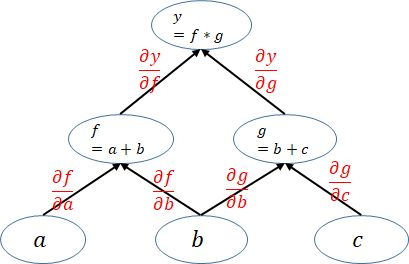图的细节

pytorch构建的计算图是动态图，为了节约内存，所以每次一轮迭代完之后计算图就被在内存释放，所以当你想要多次backward时候就会报如下错：

net = nn.Linear(3, 4)  # 一层的网络，也可以算是一个计算图就构建好了 input = torch.randn(2, 3, requires_grad=True)  # 定义一个图的输入变量 output = net(input)  # 最后的输出 loss = torch.sum(output)  # 这边加了一个sum() ,因为被backward只能是标量 loss.backward() # 到这计算图已经结束，计算图被释放了

net = nn.Linear(3, 4) input = torch.randn(2, 3, requires_grad=True) output = net(input) loss = torch.sum(output)  loss.backward() loss.backward()  RuntimeError: Trying to backward through the graph a second time, but the buffers have already been freed.

net = nn.Linear(3, 4) input = torch.randn(2, 3, requires_grad=True) output = net(input) loss = torch.sum(output) loss.backward(retain_graph=True) # 添加retain_graph=True标识，让计算图不被立即释放 loss.backward()

x = torch.FloatTensor([[1, 2]], requires_grad=True)  # 定义一个输入变量 y = torch.FloatTensor([[3, 4],                                [5, 6]]) loss = torch.mm(x, y)    # 变量之间的运算 loss.backward(torch.FloatTensor([[1, 0]]), retain_graph=True)  # 求梯度，保留图                                     print(x.grad.data)   # 求出 x_1 的梯度 x.grad.data.zero_()  # 最后的梯度会累加到叶节点，所以叶节点清零 loss.backward(torch.FloatTensor([[0, 1]]))   # 求出 x_2的梯度 print(x.grad.data)        # 求出 x_2的梯度

3  5 [torch.FloatTensor of size 1x2]  4  6 [torch.FloatTensor of size 1x2]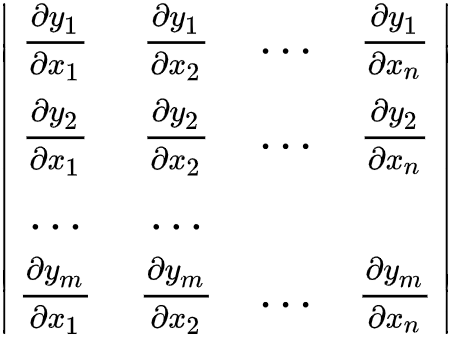RuntimeError: grad can be implicitly created only for scalar outputs

loss.backward(torch.tensor([[1.0,1.0,1.0,1.0]]))

loss.backward(torch.tensor([[0.1,1.0,10.0,0.001]]))

Tensor的自动求导的技巧

保存在backward时自动抛弃的中间变量梯度

# hook(module, input, output) -> None or modified output # input 是输入单元的数据，output 是单元处理输出后的结果 register_forward_hook(hook)  # hook(module, grad_input, grad_output) -> Tensor or None register_backward_hook(hook)  # hook(module, input) -> None or modified input register_forward_pre_hook(hook)

detach 函数的作用

x=T.ones(10, requires_grad=True) y=x*2 z=x*3 r=(y+z).sum() make_dot(r).render("attached", format="png")>>> r.backward() >>> x.grad tensor([5., 5., 5., 5., 5., 5., 5., 5., 5., 5.])

y=x*2 z=x.detach()*3 r=(y+z).sum() make_dot(r).render("detached", format="png")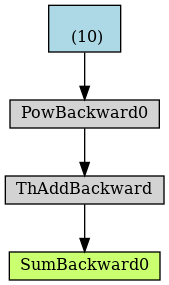>>> r.backward() >>> x.grad tensor([2., 2., 2., 2., 2., 2., 2., 2., 2., 2.])
Share